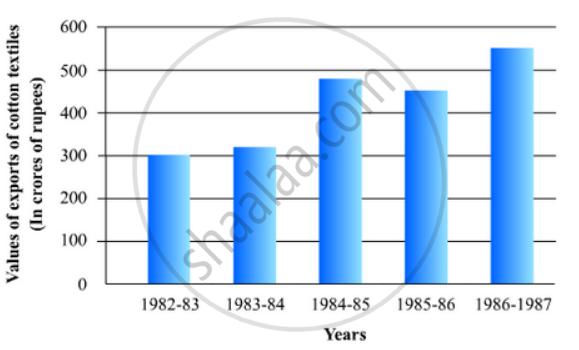# The following data gives the value (in crores of rupees) of the Indian export of cotton textiles for different years: - Mathematics

The following data gives the value (in crores of rupees) of the Indian export of cotton textiles for different years:

 Years 1982 1983-1984 1984-1985 1985-1986 1986-1987 Value of Exportof Cotton Textiles(in crores of rupees) 300 325 475 450 550

Represent the above data with the help of a bar graph. Indicate with the help of a bar graph the year in which the rate of increase in exports is maximum over the preceding year.

#### Solution

To represent the given data by a vertical bar graph, we first draw horizontal and vertical axes. Let us consider that the horizontal and vertical axes represent the years and the value of Indian export of cotton textiles in Crores of rupees respectively. We have to draw 5 bars of different lengths given in the table.

At first we mark 5 points in the horizontal axis at equal distances and erect rectangles of the same width at these points. The heights of the rectangles are proportional to the values of Indian export of cotton textiles in different years.

The vertical bar graph of the given data is as follows:The export increases in the years 1983-84, 1984-85 and 1986-87. Now,

(a) The rate of increase in the year 1983-84 is

(325-300)/300 xx 100

=25/3

= 8.33%

(b) The rate of increase in the year 1984-85 is

(475-325)/325 xx 100

=15000/325

600/13

= 46.15%

(c) The rate of increase in the year 1986-87 is

(550-450)/450 xx 100

= 10000/450

=22.22%

Hence, in the year 1984-85 the rate of increase of export is the maximum over the preceding year.

Concept: Graphical Representation of Data
Is there an error in this question or solution?

#### APPEARS IN

RD Sharma Mathematics for Class 9
Chapter 23 Graphical Representation of Statistical Data
Exercise 23.2 | Q 15 | Page 27

Share